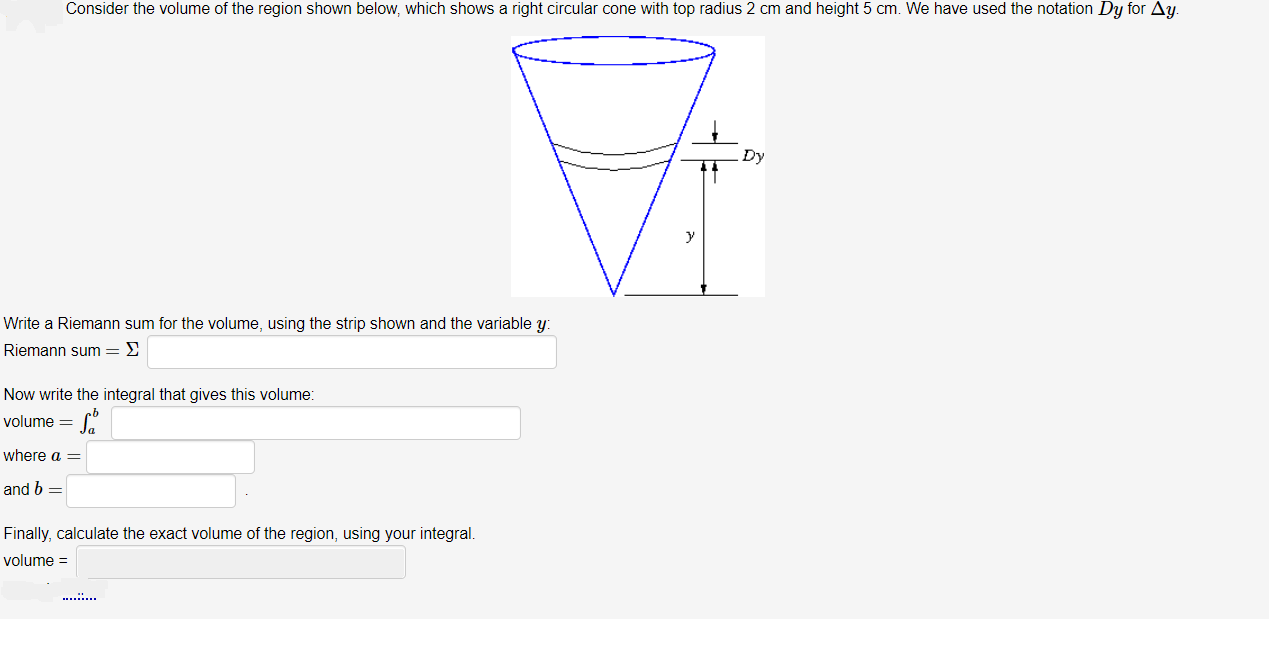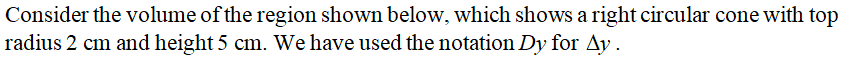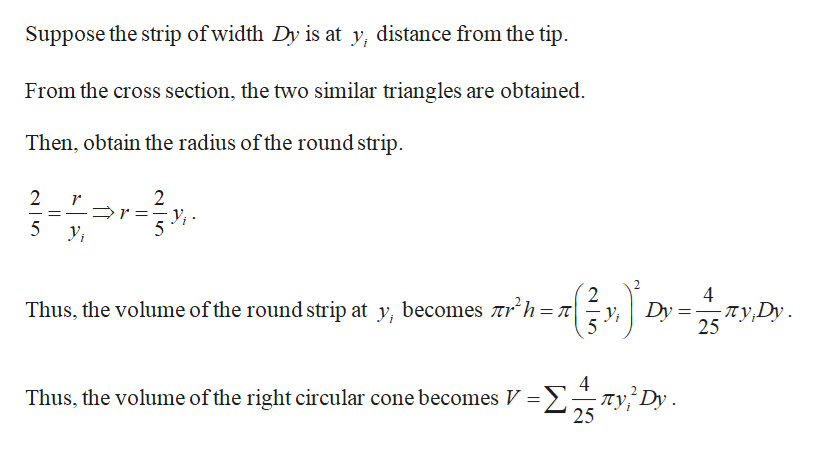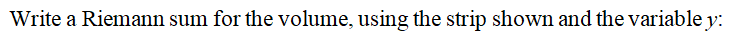# Consider the volume of the region shown below, which shows a right circular cone with top radius 2 cm and height 5 cm. We have used the notation Dy for Ay.DyWrite a Riemann sum for the volume, using the strip shown and the variable y:Riemann sum = 2Now write the integral that gives this volume:volume =where aand b =Finally, calculate the exact volume of the region, using your integral.volume =

Question
30 viewshelp_outlineImage TranscriptioncloseConsider the volume of the region shown below, which shows a right circular cone with top radius 2 cm and height 5 cm. We have used the notation Dy for Ay. Dy Write a Riemann sum for the volume, using the strip shown and the variable y: Riemann sum = 2 Now write the integral that gives this volume: volume = where a and b = Finally, calculate the exact volume of the region, using your integral. volume = fullscreen
check_circle

Step 1help_outlineImage TranscriptioncloseSuppose the strip of width Dy is at y, distance from the tip. From the cross section, the two similar triangles are obtained. Then, obtain the radius of the round strip. 4 -Ty,Dy. 25 Thus, the volume of the round strip at y, becomes tr'h=T| Y; Dy 4 пу, 25 Σ Τ Dy . Thus, the volume of the right circular cone becomes V fullscreen
Step 2...

### Want to see the full answer?

See Solution

#### Want to see this answer and more?

Solutions are written by subject experts who are available 24/7. Questions are typically answered within 1 hour.*

See Solution
*Response times may vary by subject and question.
Tagged in

### Integration